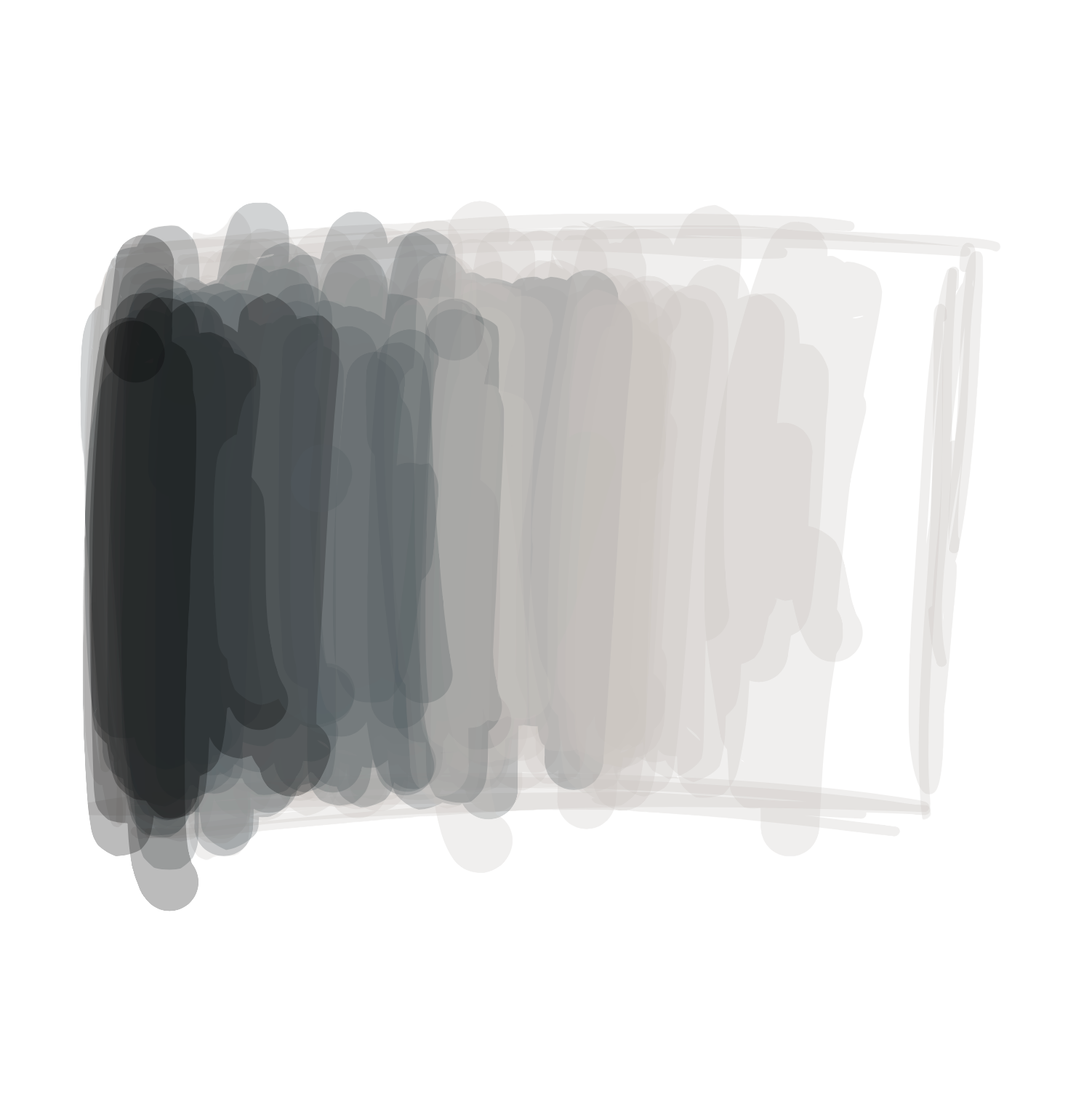# Placing things on the screen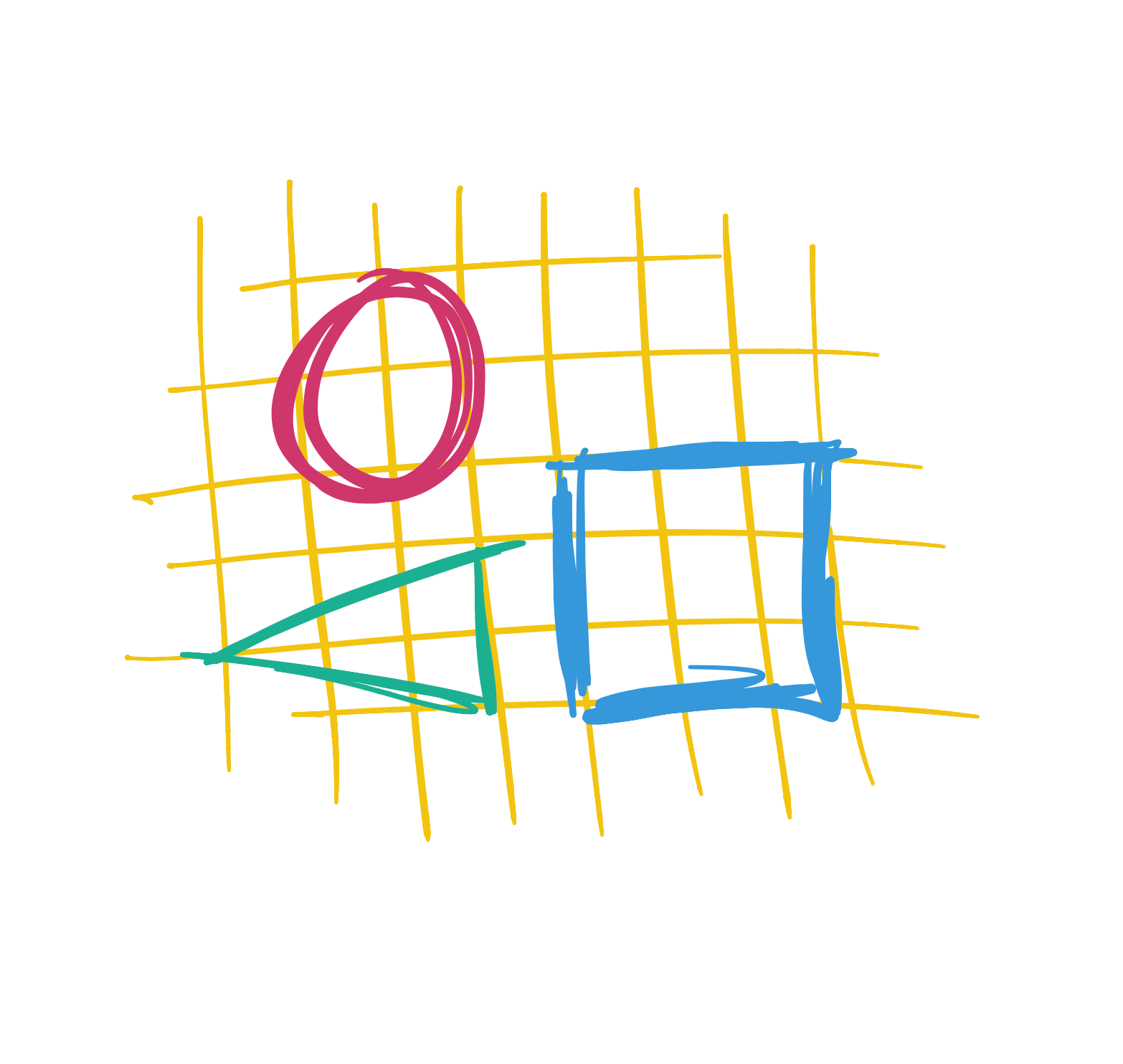So far, we’ve written a number of functions - like `ellipse` - with a bunch of numeric arguments - such as `(10,10,10,10)` - that tell the program where to put the shapes and how big to make them. But what do these numbers mean?

The screen you see in front of you is made up of millions of teeny, tiny lights. When we write a program, we tell the computer what to do with these lights. But in order to do this, we need a way to tell the computer which lights we want to control.

If you hold a magnifying glass in front of a screen, you will see that it looks a little bit like this: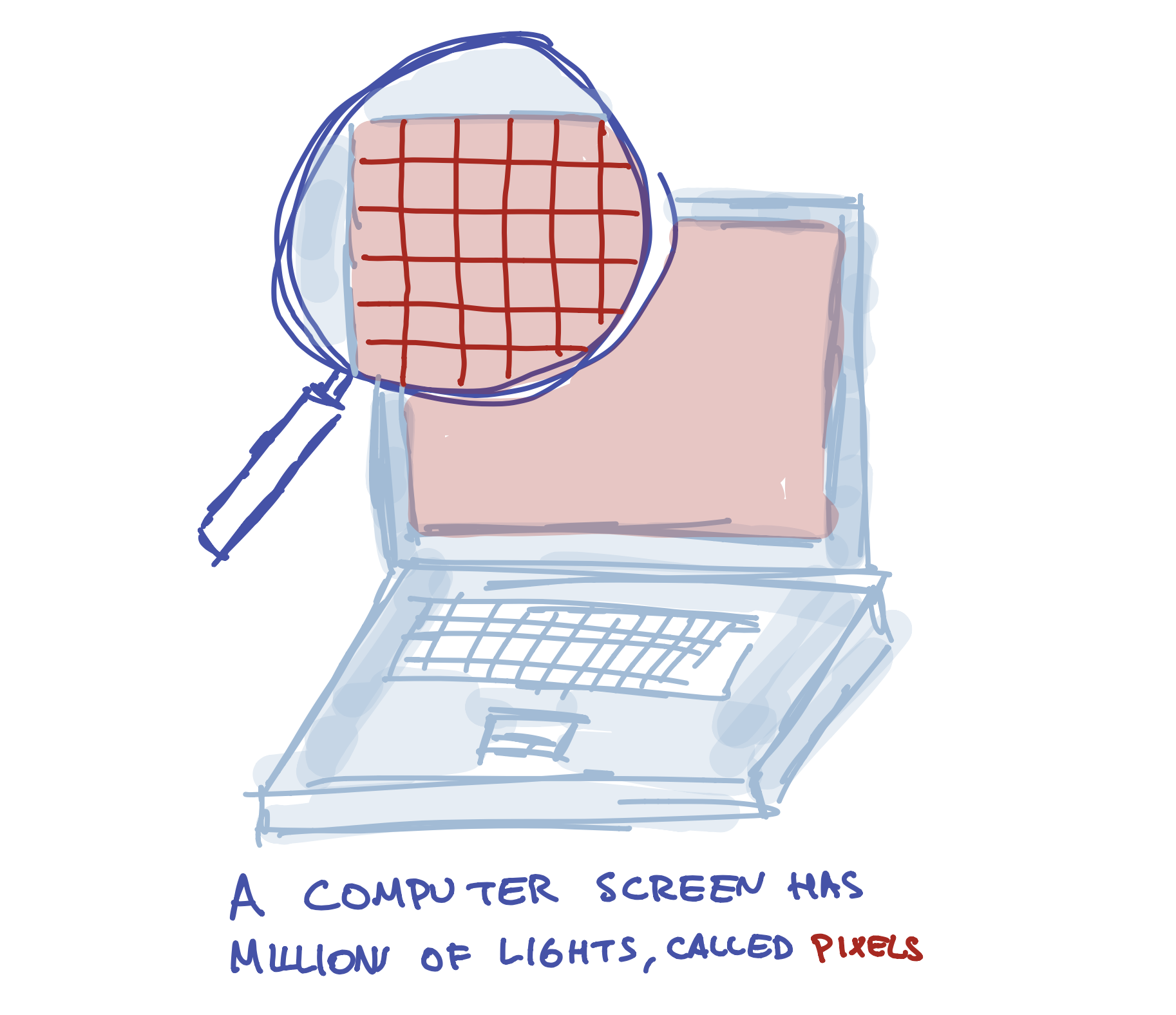One way to think of a screen is like a graph paper: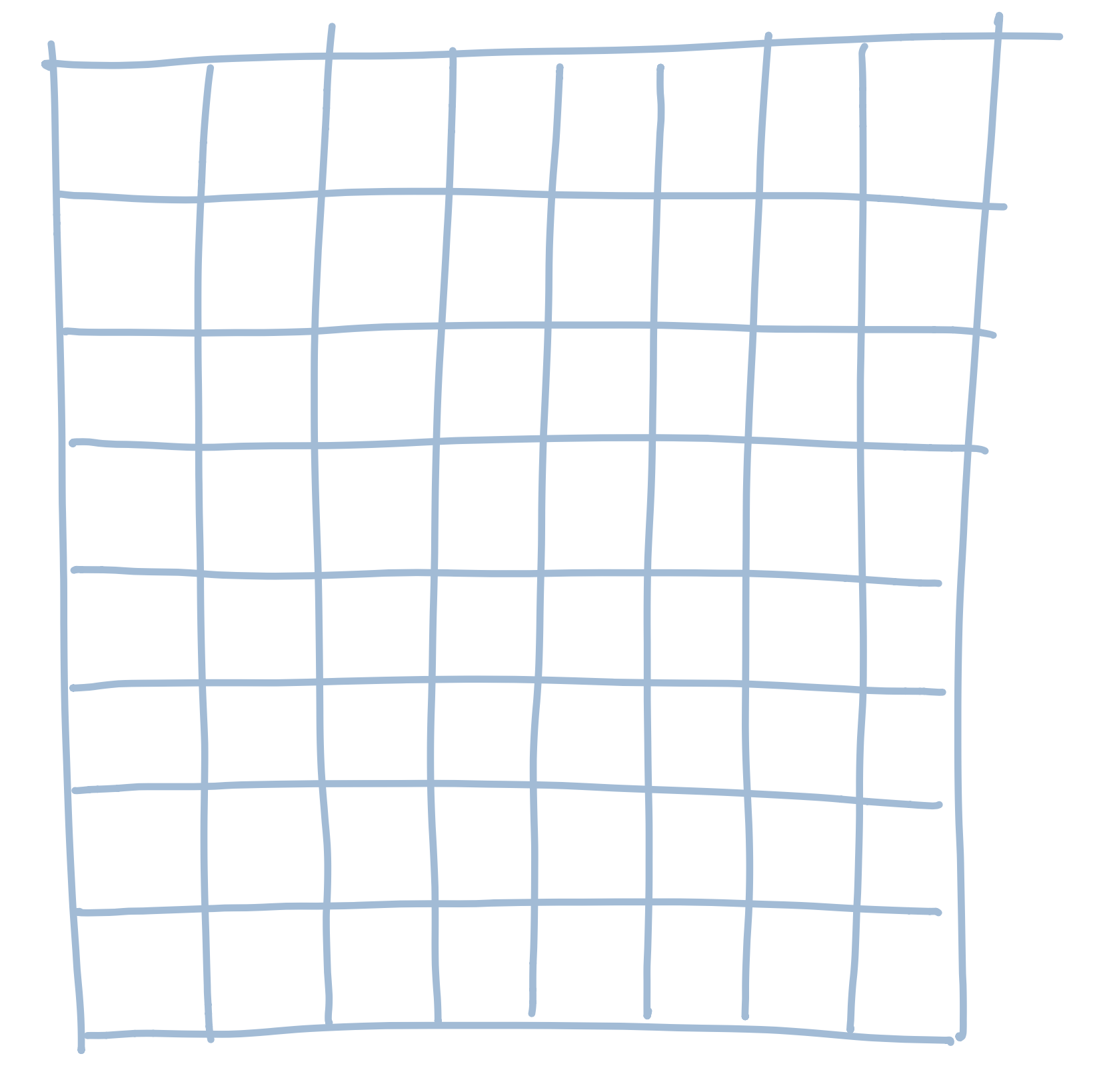We need to pick a number for each light. The easiest way to do this (and the way programs do it) is by starting from the left side of the screen and giving the first column the number 1. We give the second column the number 2. The third is 3, and so on. So it looks a bit like this: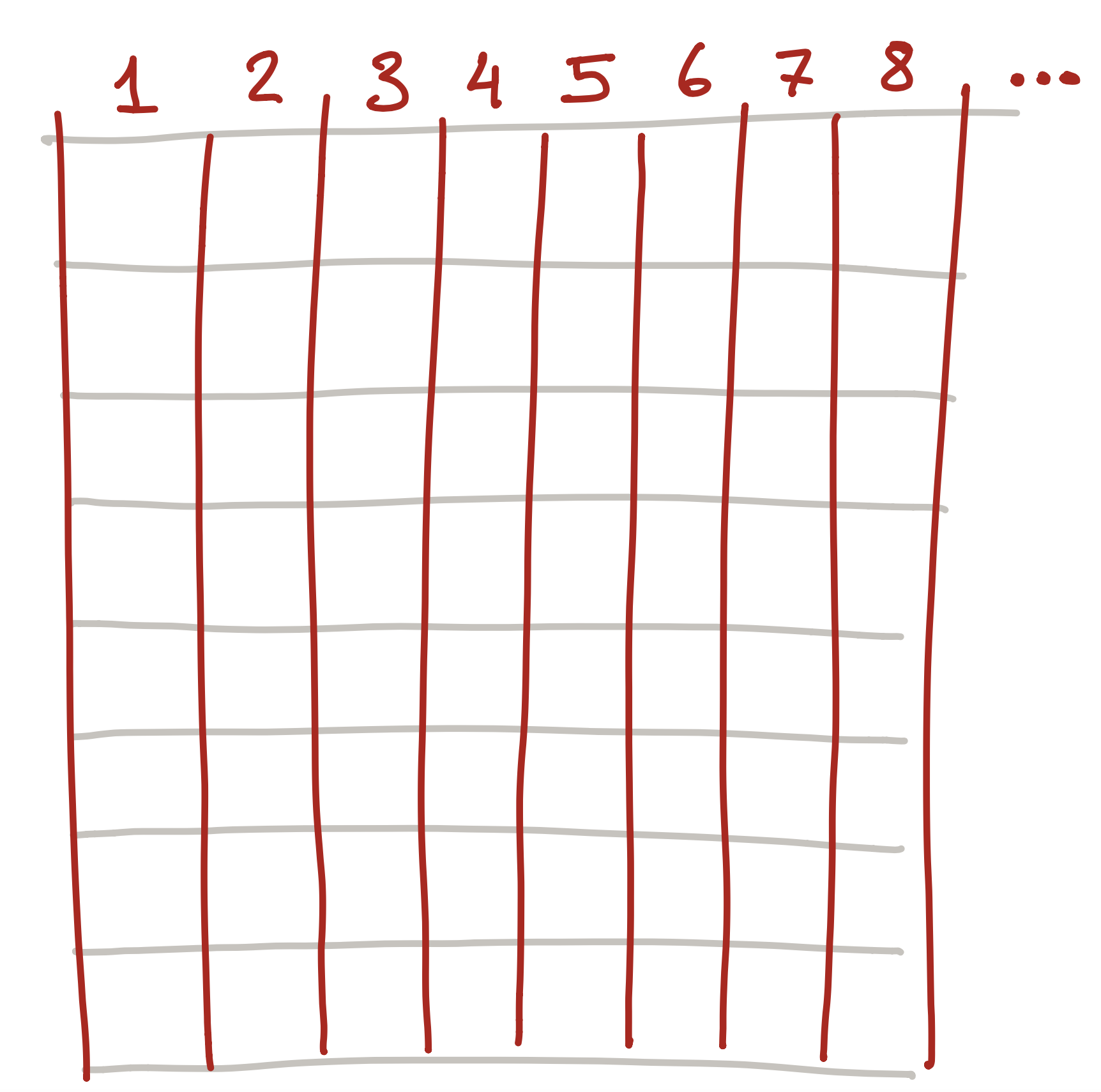But this isn’t quite good enough! We can pick the column we want to control, such as column 3: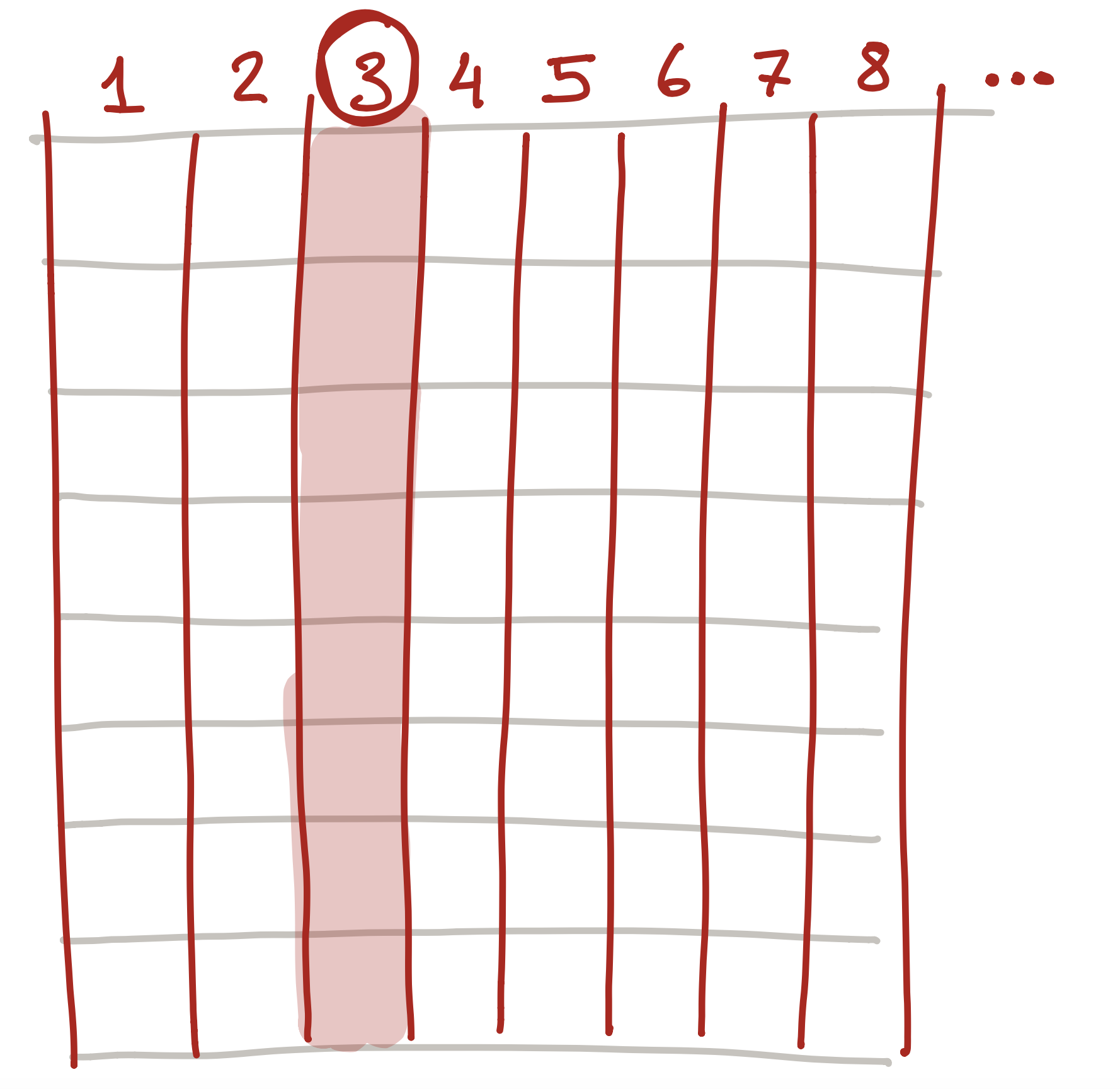But what if we want to control a specific light? To do this, we also number each row starting from the top: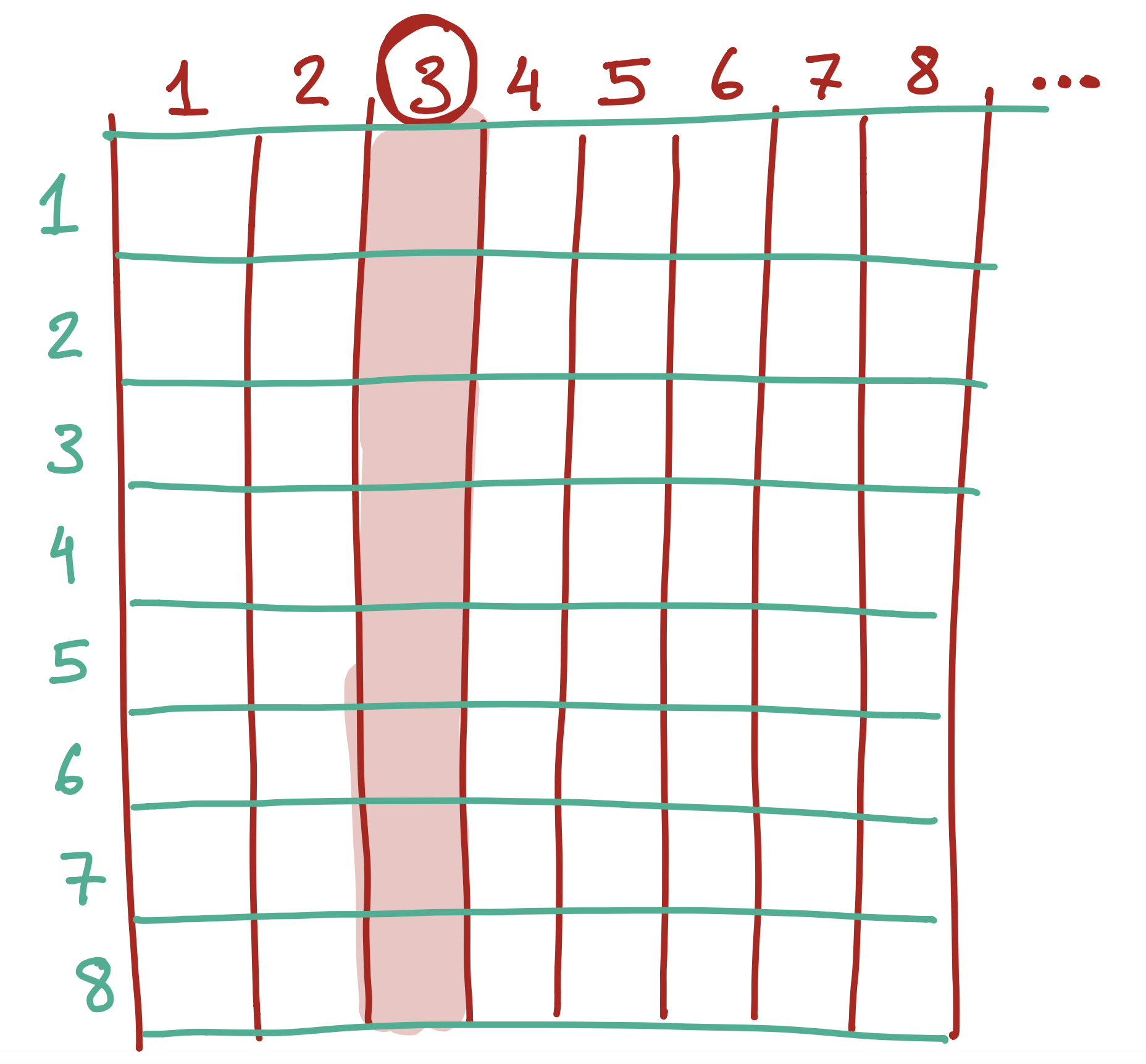So each light actually has two numbers - the column and the row. So, the number in black below…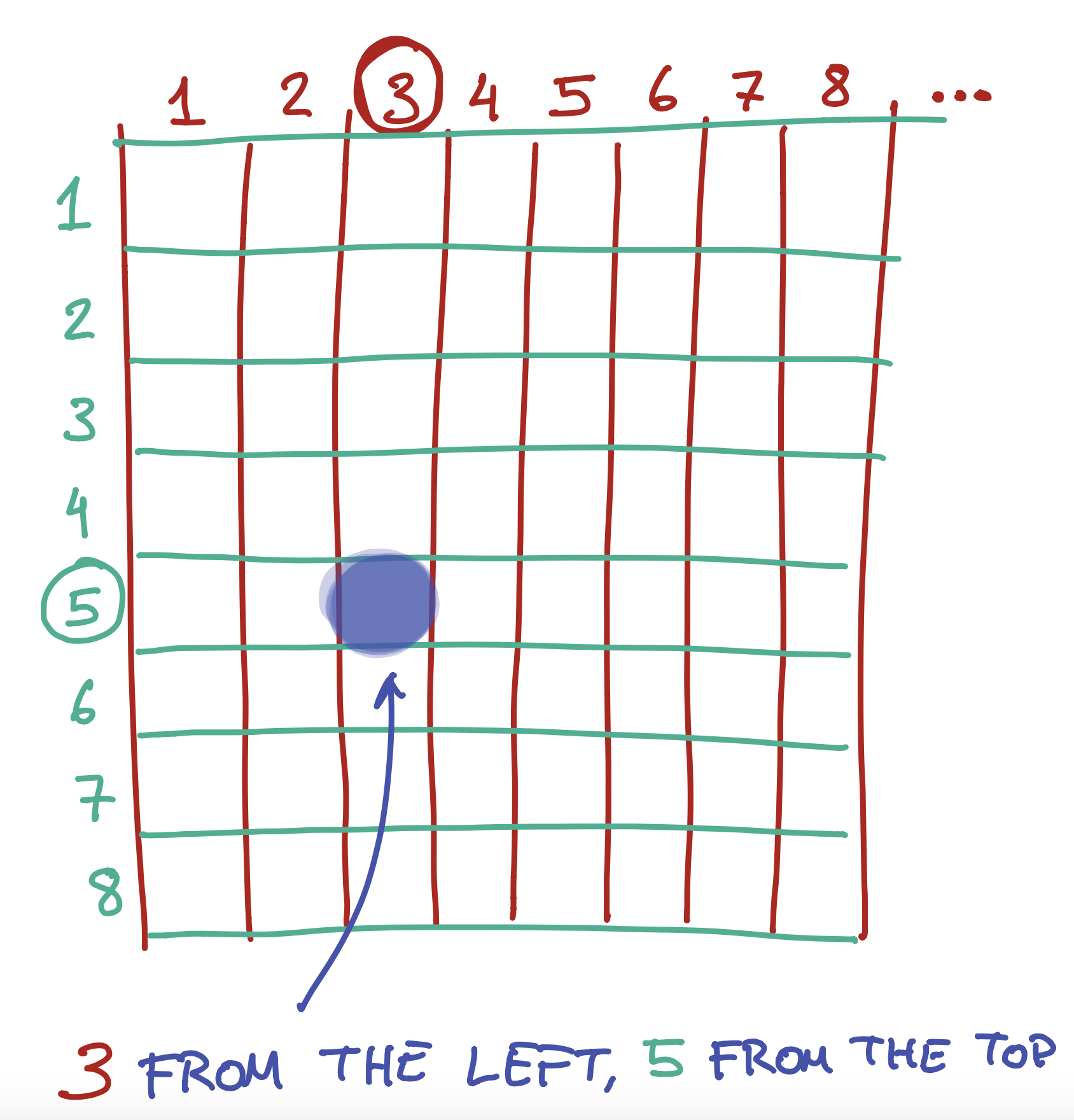..is in the 3rd colummn from the left, and the 2nd column from the top.

To do this, we give each light a number. Actually, we give it two - a number from the left, and a number from the top.

We can also use the same numbers to tell the computer how wide or tall to make something. So, for example, you could say “draw a rectangle that starts from the 3rd light on the left and the 5th light on the top, and make it 5 lights wide and 3 lights tall”.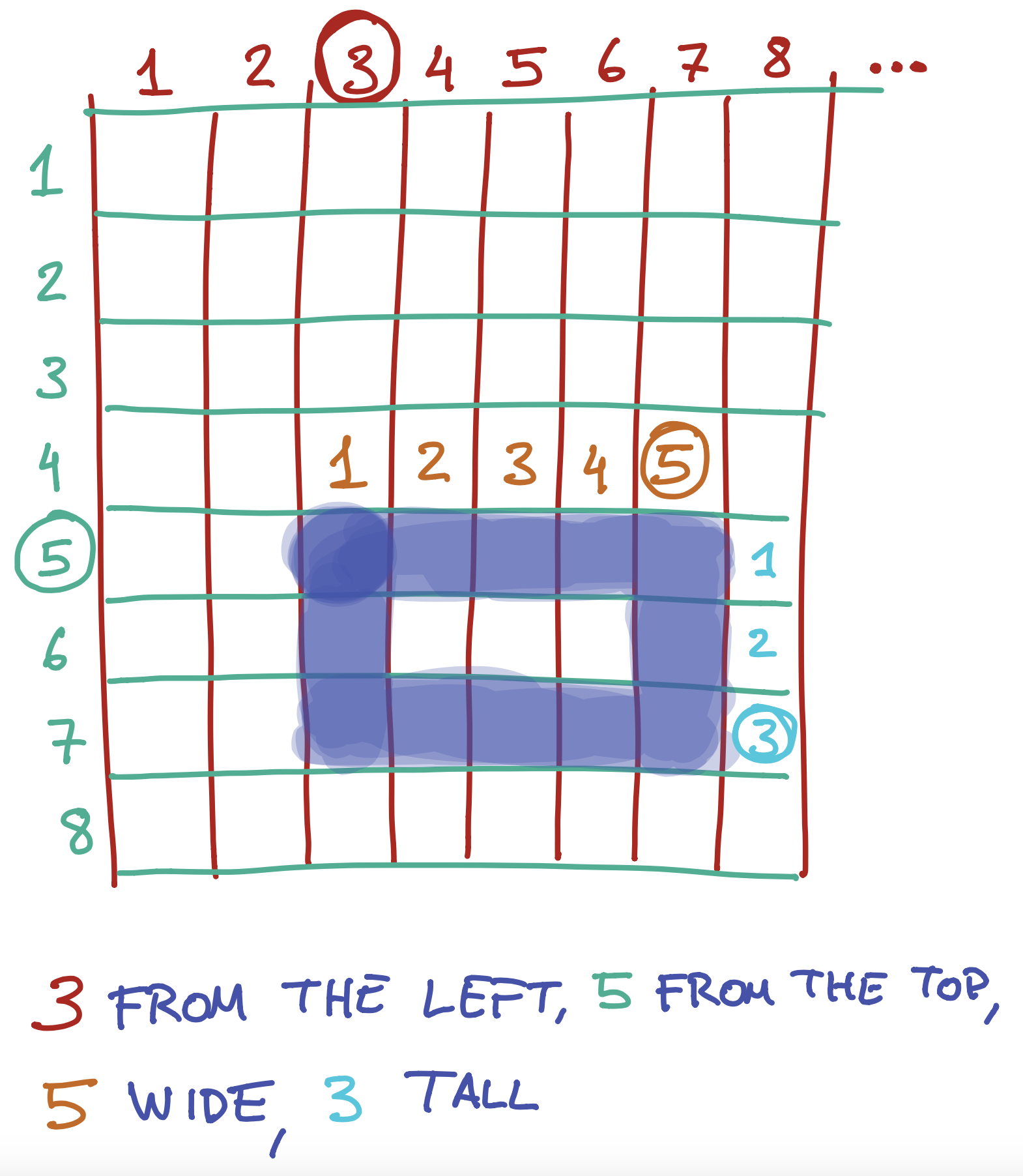This is exactly what we do when we write the following instruction:

##### Code:
```rect(3,5,5,3);
```
##### Preview:

The four arguments we give the `rect` function are instructions on where to start the rectangle on the left and top, and how wide and tall to make it: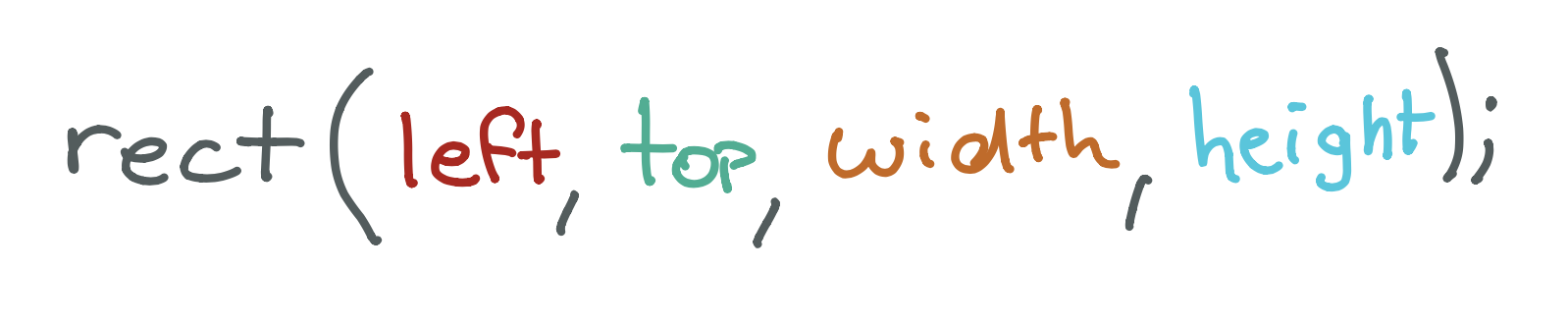Exercise

What would the four (left, top, width, height) arguments for this rectangle be?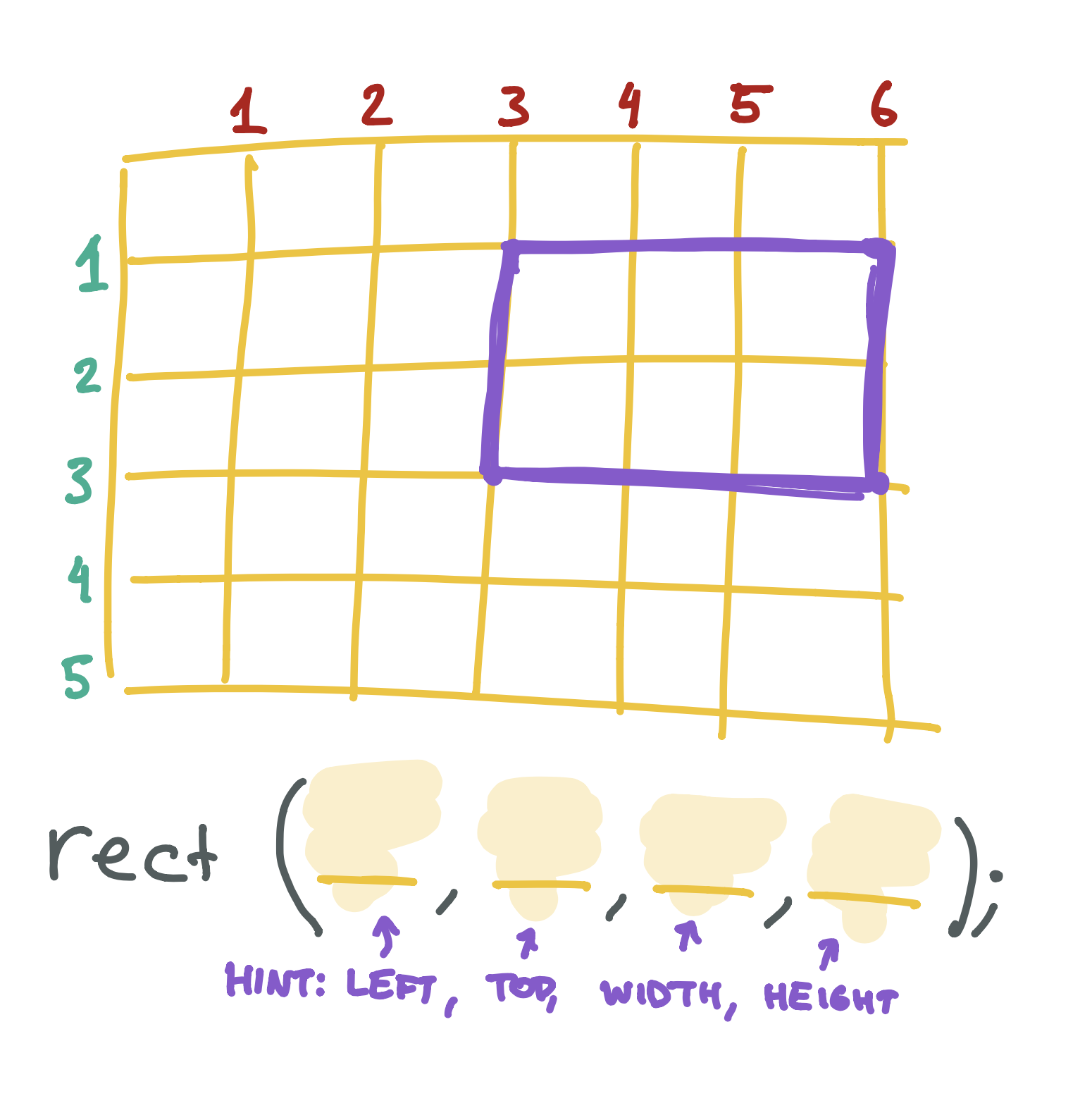Exercise

Draw your own rectangle on the graph paper below, then write the rect function that would create it.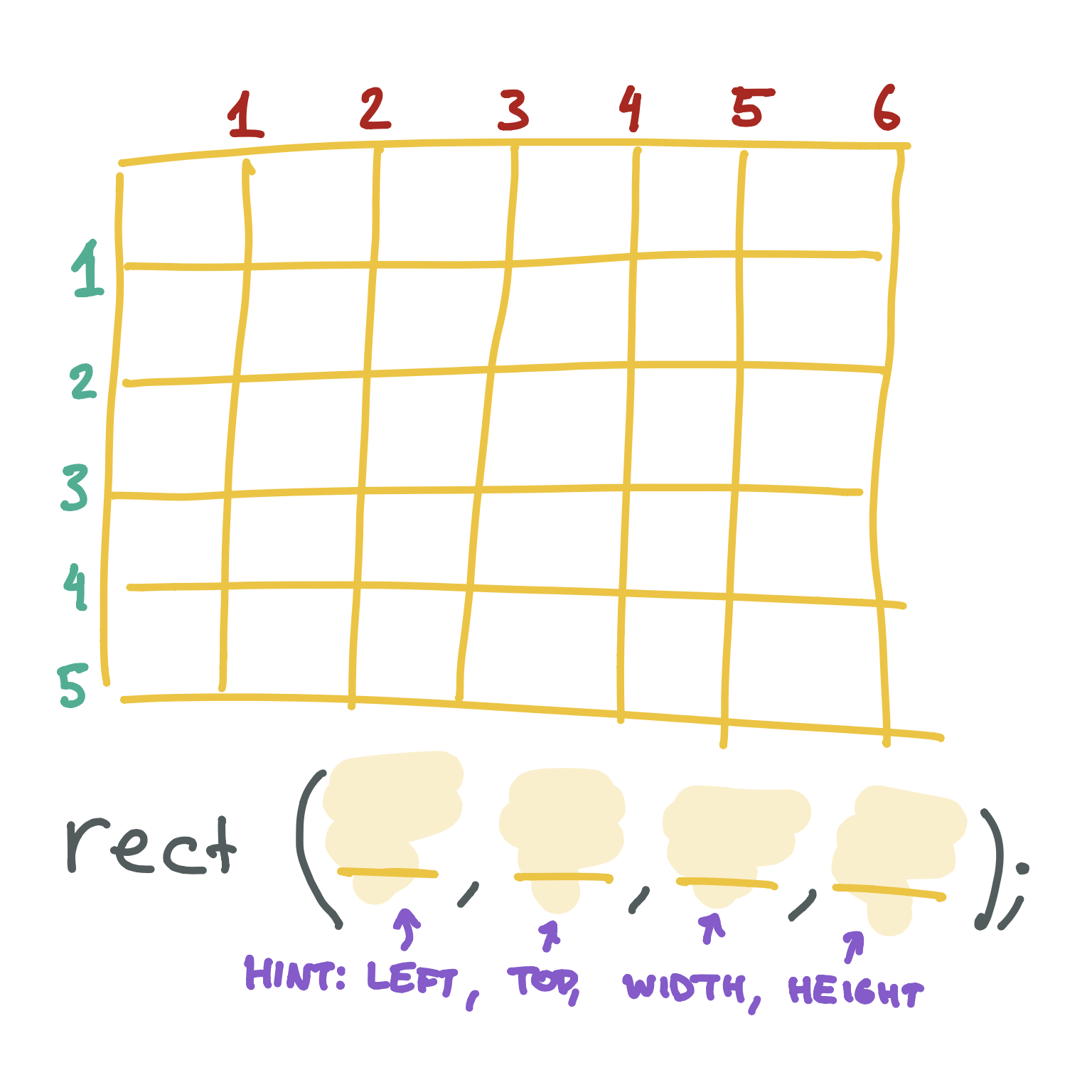## X & Y

We generally don’t use the words left and top when we are talking about where something is on the screen. Instead, we normally use the letter x to talk about the left-to-right part and y to talk about the top-to-bottom part: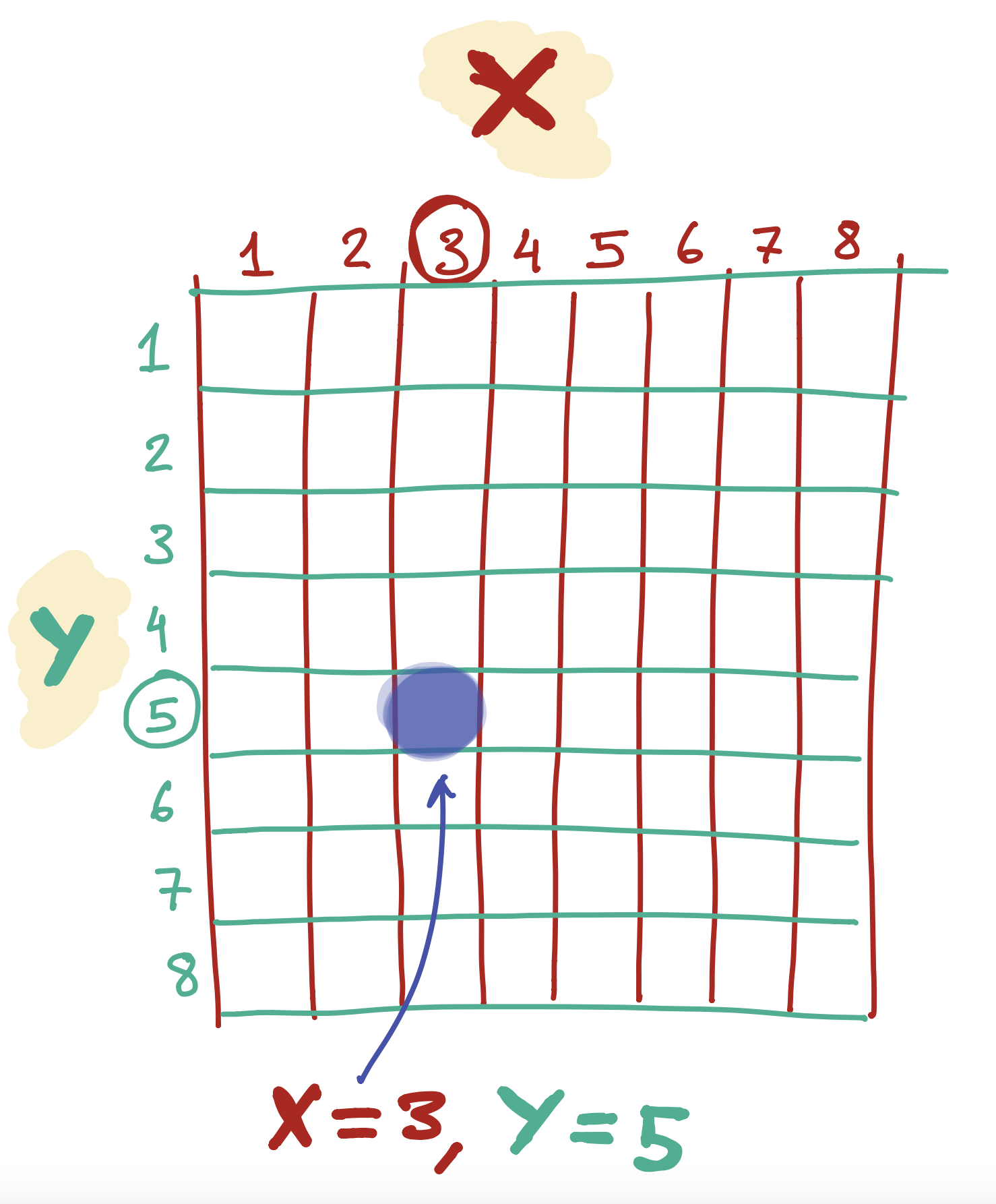If you’ve done any math homework with shapes, you may have seen these letters before!

## Placing a line on the screen

The line function is a little different from the rect function. To draw a line, we pick two points on the screen and tell the computer to make a line from one to the other.

The first two arguments are the left & top values for the first point - we generally call this x1 and y1. The next two arguments are the left & top values for the second point - we generally call this x2 and y2: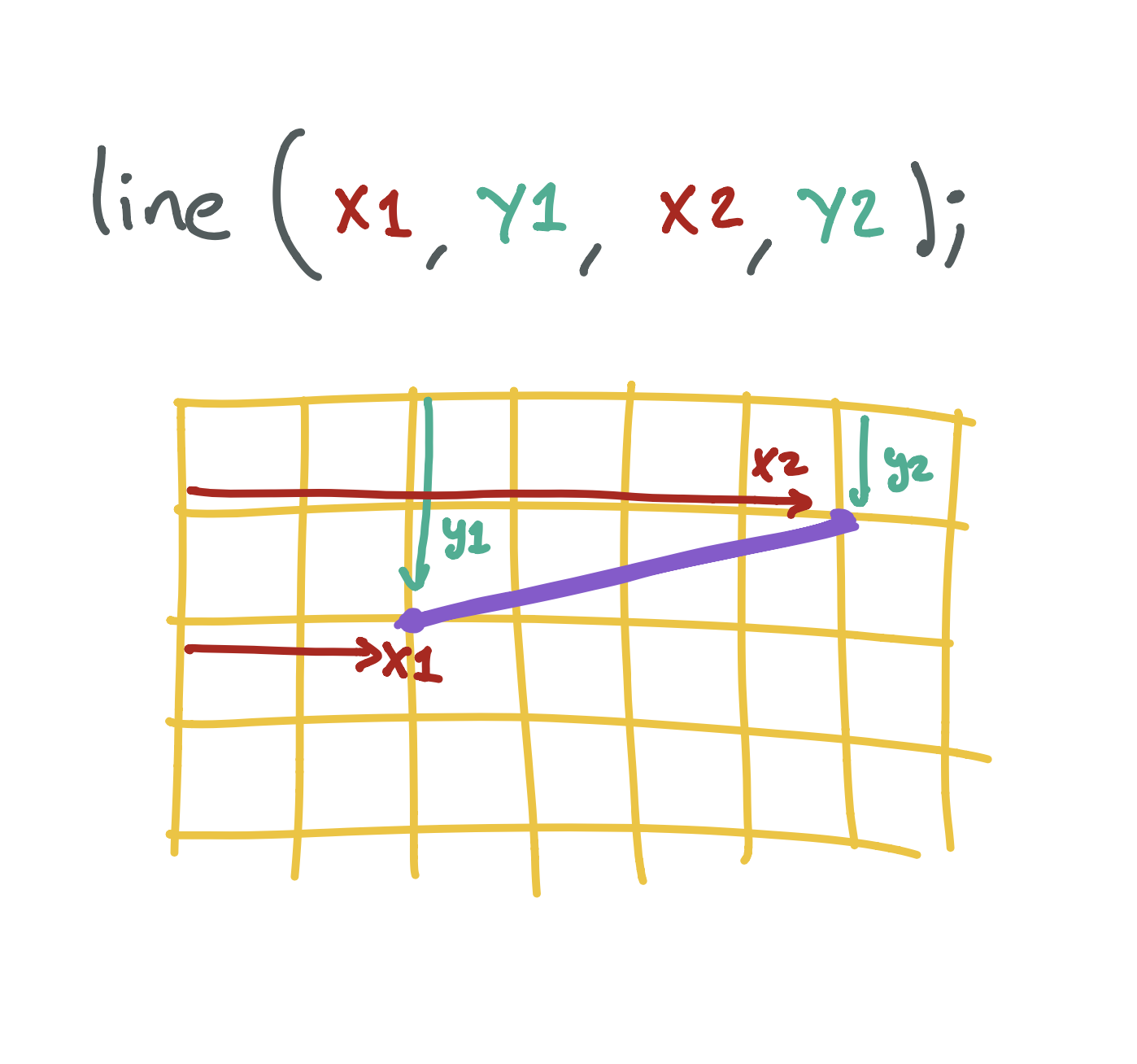## Placing an ellipse/circle on the screen

The ellipse function is a little different, because it doesn’t have a top and left point - circles don’t really have an edge we can use for this!

Instead, we tell the program where to put the middle of the circle from the left and top of the screen (we call this center x and center y), as well as how wide and tall to make it: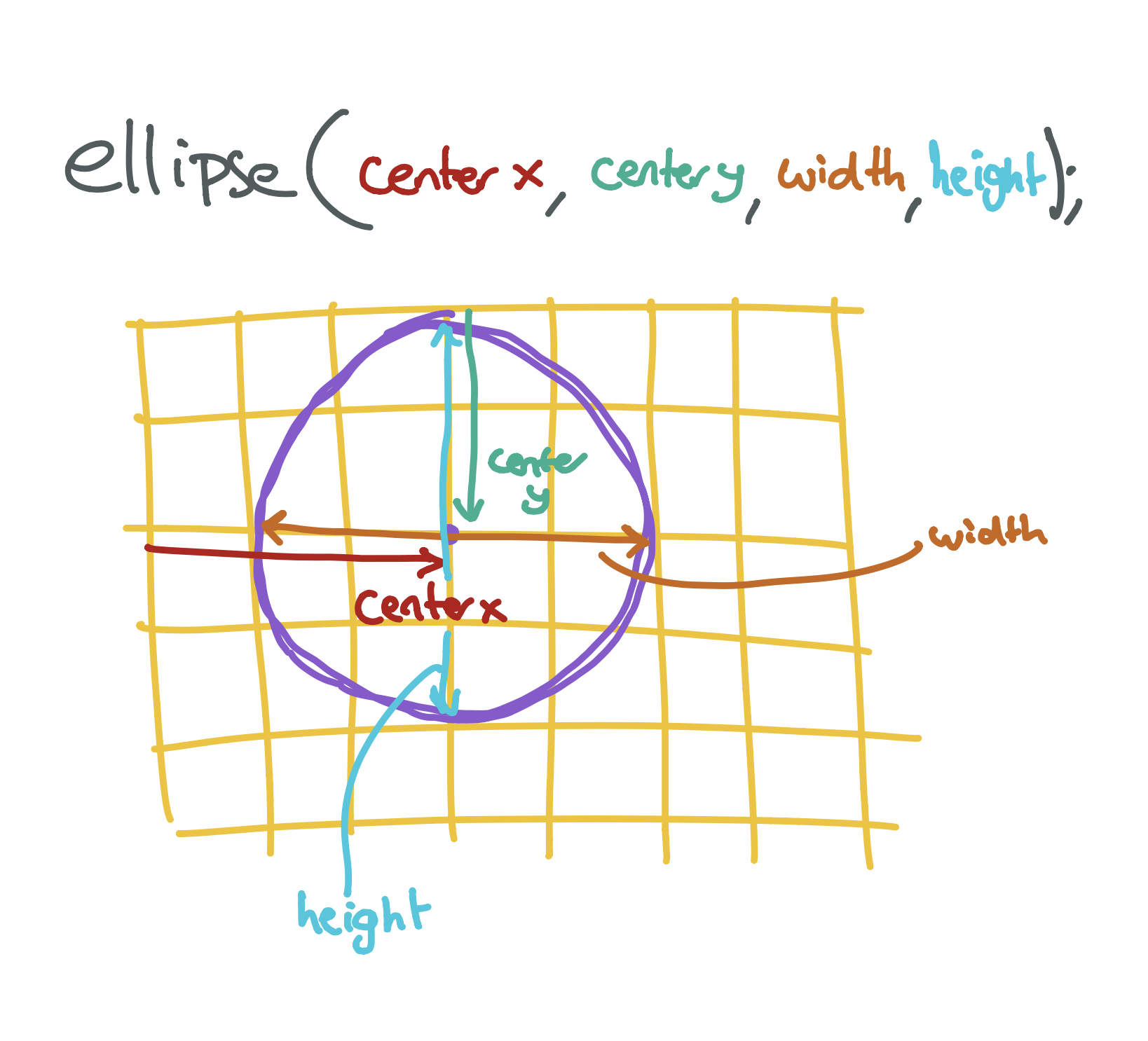## Placing a triangle on the screen

The triangle function is the weirdest! Triangles have three points, and so we need to tell the program where to put each of them.

The first two arguments tell the program where to put the first point from the left and top of the screen (we call this x1 and y1), the second two arguments tell us where to put the second point from the left and top of the screen (we call this x2 and y2), and the last two arguments tell us where to put the third point from the left and top of the screen (we call this x3 and y3):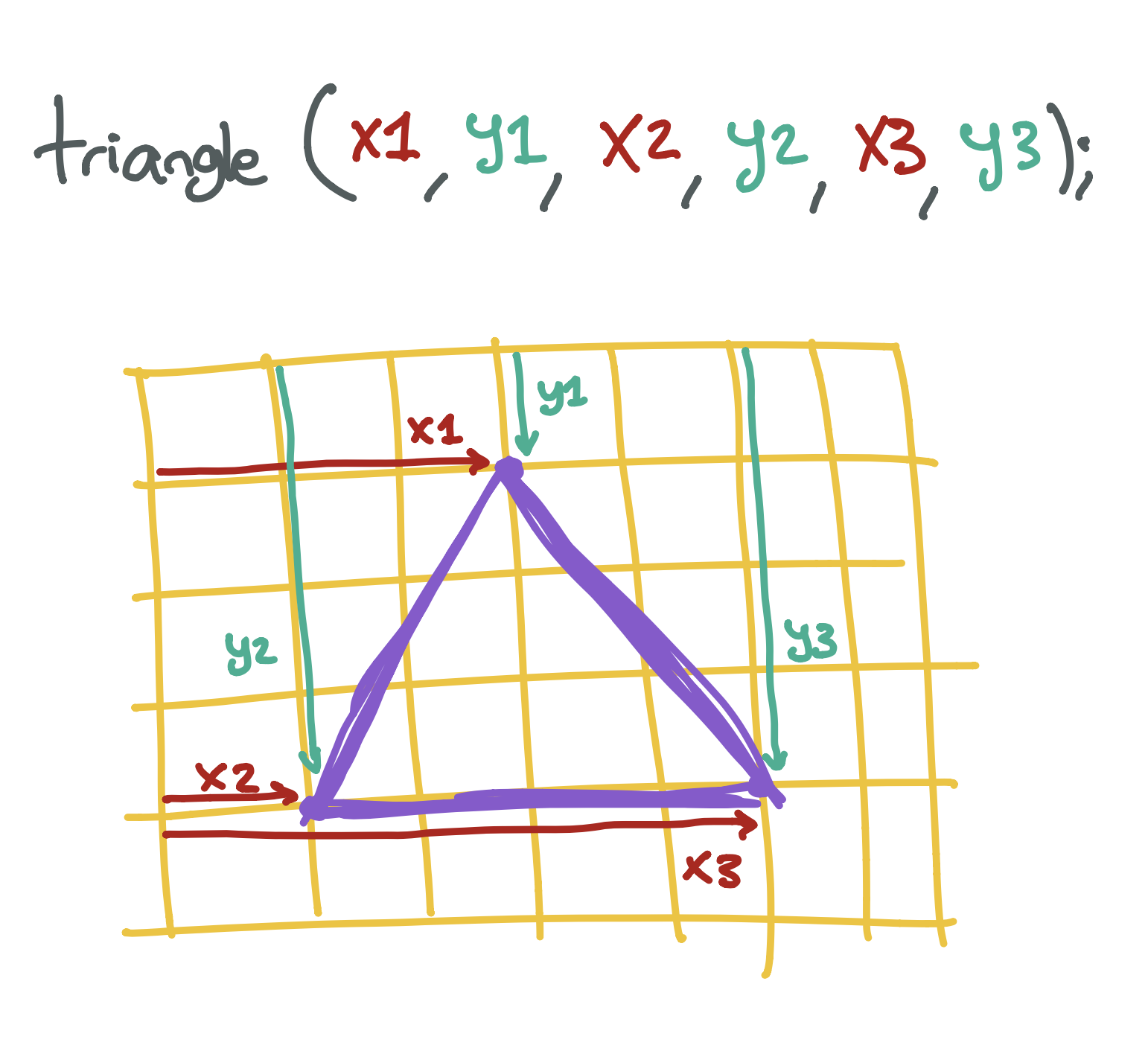Tip

Don’t worry if you are having a hard time figuring out which numbers to write down! A lot of programmers don’t know what they are doing when they type in the numbers. You can always try random numbers until you find something that looks like what you want. The more you play around, the more your brain will be able to guess what the numbers should be..

Exercise

Create a program that draws a face on the screen. You will need to draw two circles - one for each eye - and a rectangle for the mouth. If you want, you can also draw a triangle for the nose!

Next lesson Black, White, and In Between